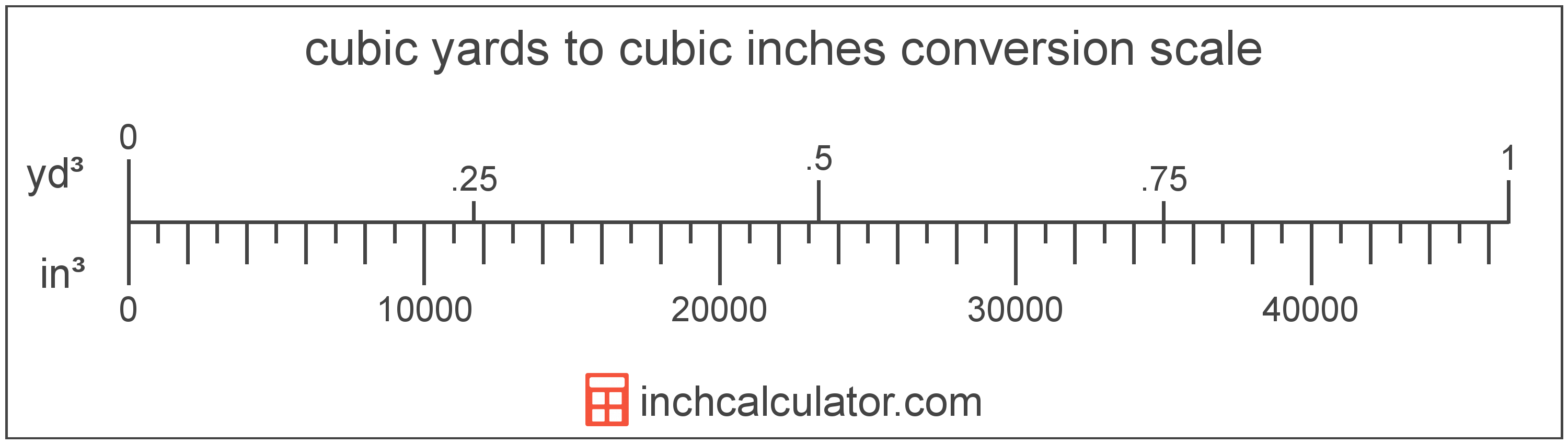# Cubic Yards to Cubic Inches Conversion

Enter the volume in cubic yards below to get the value converted to cubic inches.

(find cubic yards)
Results in Cubic Inches:1 yd³ = 46,656.000733 in³

## How to Convert Cubic Yards to Cubic InchesTo convert a cubic yard measurement to a cubic inch measurement, multiply the volume by the conversion ratio.

Since one cubic yard is equal to 46,656.000733 cubic inches, you can use this simple formula to convert:

cubic inches = cubic yards × 46,656.000733

The volume in cubic inches is equal to the cubic yards multiplied by 46,656.000733.

For example, here's how to convert 5 cubic yards to cubic inches using the formula above.
5 yd³ = (5 × 46,656.000733) = 233,280.003666 in³

## Cubic Yards

The cubic yard is a unit of volume that is equal to the space consumed by a cube with each edge measuring one yard.

The cubic yard is a US customary and imperial unit of volume. A cubic yard is sometimes also referred to as a cubic yd. Cubic yards can be abbreviated as yd³, and are also sometimes abbreviated as cu yard, cu yd, or CY. For example, 1 cubic yard can be written as 1 yd³, 1 cu yard, 1 cu yd, or 1 CY.

You might also be interested in our cubic yards calculator.

## Cubic Inches

A cubic inch is a unit of volume equal to the space consumed by a cube with sides that are one inch in all directions. One cubic inch is equivalent to about 16.387 cubic centimeters or 0.554 fluid ounces.

The cubic inch is a US customary and imperial unit of volume. A cubic inch is sometimes also referred to as a cubic in. Cubic inches can be abbreviated as in³, and are also sometimes abbreviated as cu inch, cu in, or CI. For example, 1 cubic inch can be written as 1 in³, 1 cu inch, 1 cu in, or 1 CI.

## Cubic Yard to Cubic Inch Conversion Table

Cubic yard measurements converted to cubic inches
Cubic Yards Cubic Inches
0.0001 yd³ 4.6656 in³
0.0002 yd³ 9.3312 in³
0.0003 yd³ 14 in³
0.0004 yd³ 18.66 in³
0.0005 yd³ 23.33 in³
0.0006 yd³ 27.99 in³
0.0007 yd³ 32.66 in³
0.0008 yd³ 37.32 in³
0.0009 yd³ 41.99 in³
0.001 yd³ 46.66 in³
0.002 yd³ 93.31 in³
0.003 yd³ 139.97 in³
0.004 yd³ 186.62 in³
0.005 yd³ 233.28 in³
0.006 yd³ 279.94 in³
0.007 yd³ 326.59 in³
0.008 yd³ 373.25 in³
0.009 yd³ 419.9 in³
0.01 yd³ 466.56 in³
0.02 yd³ 933.12 in³
0.03 yd³ 1,400 in³
0.04 yd³ 1,866 in³
0.05 yd³ 2,333 in³
0.06 yd³ 2,799 in³
0.07 yd³ 3,266 in³
0.08 yd³ 3,732 in³
0.09 yd³ 4,199 in³
0.1 yd³ 4,666 in³
0.2 yd³ 9,331 in³
0.3 yd³ 13,997 in³
0.4 yd³ 18,662 in³
0.5 yd³ 23,328 in³
0.6 yd³ 27,994 in³
0.7 yd³ 32,659 in³
0.8 yd³ 37,325 in³
0.9 yd³ 41,990 in³
1 yd³ 46,656 in³

## References

1. National Institute of Standards and Technology, Specifications, Tolerances, and Other Technical Requirements for Weighing and Measuring Devices, Handbook 44 - 2019 Edition, https://nvlpubs.nist.gov/nistpubs/hb/2019/NIST.HB.44-2019.pdf